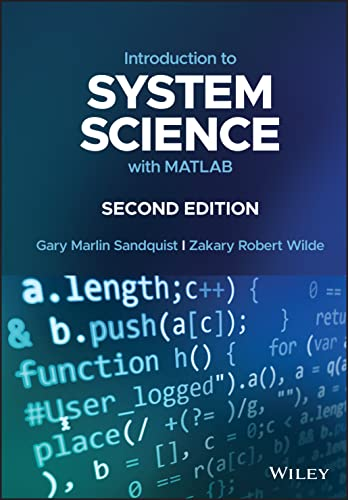# Introduction to System Science with MATLAB, 2nd Edition

• Length: 512 pages
• Edition: 2
• Publisher:
• Publication Date: 2023-01-12
• ISBN-10: 1119213967
• ISBN-13: 9781119213963
Description

Introduction to SYSTEM SCIENCE with MATLAB

Explores the mathematical basis for developing and evaluating continuous and discrete systems

In this revised Second Edition of Introduction to System Science with MATLAB®, the authors Gary Sandquist and Zakary Wilde provide a comprehensive exploration of essential concepts, mathematical framework, analytical resources, and productive skills required to address any rational system confidently and adequately for quantitative evaluation.

This Second Edition is supplemented with new updates to the mathematical and technical materials from the first edition. A new chapter to assist readers to generalize and execute algorithms for systems development and analysis, as well as an expansion of the chapter covering specific system science applications, is included.

The book provides the mathematical basis for developing and evaluating single and multiple input/output systems that are continuous or discrete. It offers the mathematical basis for the recognition, definition, quantitative modeling, analysis, and evaluation in system science.

The book also provides:

• A comprehensive introduction to system science and the principles of causality and cause and effect operations, including their historical and scientific background
• A complete exploration of fundamental systems concepts and basic system equations, including definitions and classifications
• Practical applications and discussions of single-input systems, multiple-input systems, and system modeling and evaluation
• An in-depth examination of generalized system analysis methods and specific system science applications

Perfect for upper-level undergraduate and graduate students in engineering, mathematics, and physical sciences, Introduction to System Science with MATLAB® will also earn a prominent place in libraries of researchers in the life and social sciences.